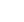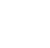# Tour the Dynamics of Change and Motion with Python

## Description

This is the third module in Engineering Computations (EngComp3), building from a foundation in Python programming to develop modeling and simulation practices, and computational problem-solving. You learn to capture motion from images and videos, to compute velocity and acceleration from position data, to obtain velocity and position from accelerometer data, and to study differential models of mechanical vibrations.   The target audience is second-year science and engineering students, with some background in calculus and ordinary differential equations. What You'll Learn Capture position data of moving objects from images and video Compute velocity and acceleration from position data, using numerical derivatives (in free-fall and projectile motion) Compute velocity and position from accelerometer data, using time-stepping to solve a differential equation Pose a second-order differential equation as a system in vector form, and compute the solution in state space Visualize direction fields and trajectories in the phase plane

## Specific details

Category of Education Technology and Engineering

## University

George Washington University

There are no comments posted here yetinfo@educom.net
 About Terms and ConditionsPrivacy PolicyRankingsAccreditations